GFG App
Open AppBrowser
Continue

# How to perform T-tests in MS Excel?

The T-Test function in Excel calculates the chance of a significant difference between two data sets, regardless of whether one or both are from the same population and have the same mean T-Test, which also includes whether the data sets we’re utilizing for computation are a one-tail or two-tail distribution with a variance that might be equal or unequal.

Formula

=T.TEST(array1, array2, tails, type)

• Array1: This is the initial group of samples being tested.
• Array2: This is the second collection of samples being compared.
• Tails: The number of tails in a distribution. There are two kinds of tails (i.e the one-tailed distribution and the two-tailed distribution)
• Type: There are three types of T-Test Function which are discussed in the following:
1. Paired: This checks to see if the mean of the paired sets is equal.
2. Equal variances: The two-sample equal variance test determines if the variance of two sets of means is equal.
3. Unequal variances: The two-sample uneven variance test determines if the variation of means between two groups is unequal.

### Method 1: Using Formula

The functioning of the T.TEST is best demonstrated by utilizing an example dataset to obtain the T.TEST’s logic.

I have classroom Test 1 and Test 2 test results. I need to run T.TEST to see whether there is a statistically significant difference between these two tests.

Sample Date: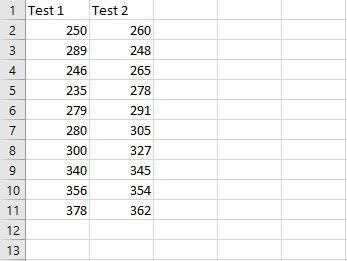Use T.TEST to determine the difference.

Example 1: Paired

The first test is a Paired two samples for a means test. In this example, we are calculating the Paired two samples for a means test using the T.TEST function taking a few arguments such as:

1. In the first parameter select the cell from A2 to A11
2. In the second parameter select the cell from B2 to B11.
3. The third parameter needs to determine how many tails the test has, it’s always best to do a two-tailed test so that’s why we are taking 2 there.
4. In the fourth parameter, we take the value as 1 for calculating the Paired two samples for a mean test.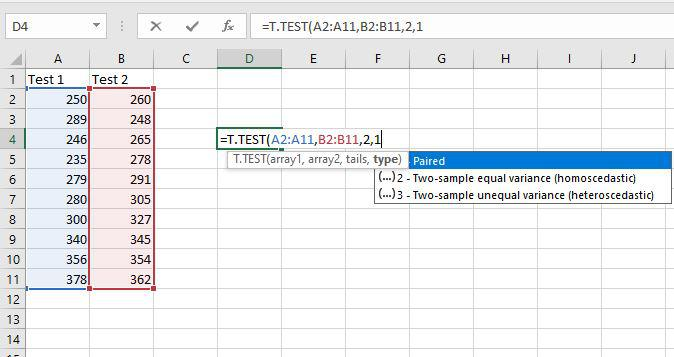The following is the outcome: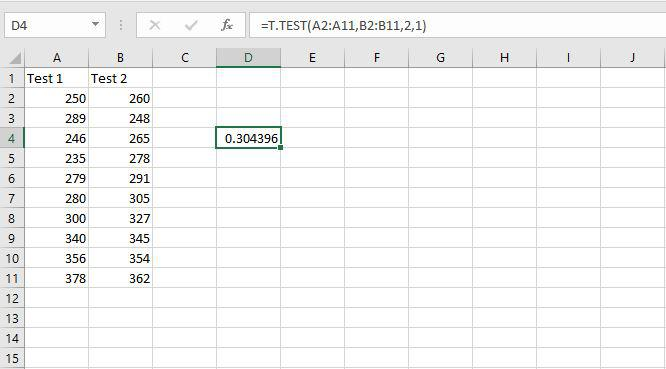Example 2: Equal variances

The second test is a Two sample assuming Equal variances test. In this example, we are calculating the Two samples assuming Equal variances test using the T.TEST function taking a few arguments such as:

1. In the first parameter select the cell from A2 to A11
2. In the second parameter select the cell from B2 to B11.
3. The third parameter needs to determine how many tails the test has, it’s always best to do a two-tailed test so that’s why we are taking 2 there.
4. In the fourth parameter, we take the value as 2 for calculating the Two samples assuming the Equal variances test.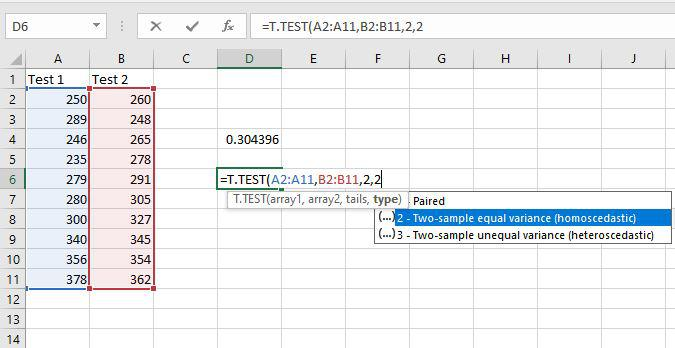The following is the outcome: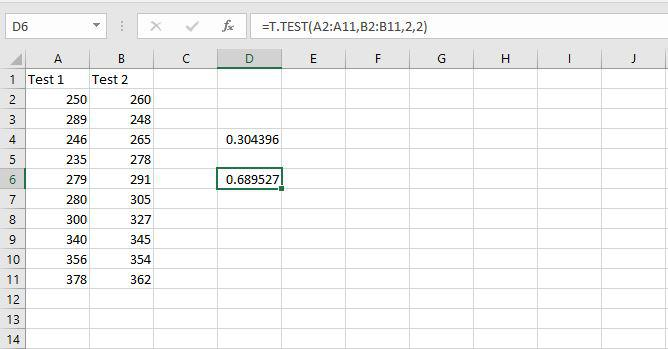Example 3: Unequal Variances

The third test is a Two-sample assuming an unequal variances test. In this example, we are calculating the Two-sample assuming an unequal variances test using the T.TEST function taking a few arguments such as:

1. In the first parameter select the cell from A2 to A11.
2. In the second parameter select the cell from B2 to B11.
3. The third parameter needs to determine how many tails the test has, it’s always best to do a two-tailed test so that’s why we are taking 2 there.
4. In the fourth parameter, we take the value as 3 for calculating the Two-sample assuming an unequal variances test.The following is the outcome: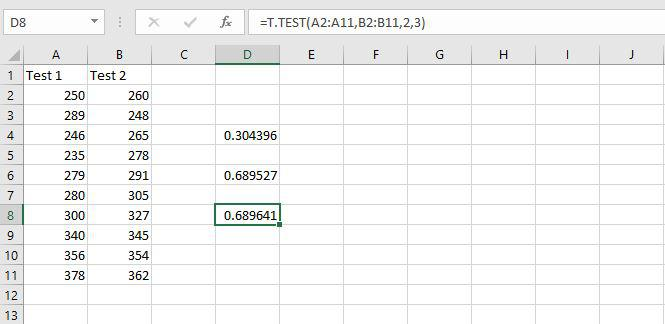The returning result is referred to as the P-value.

### Method 2: Use Analysis Tool Pack to run T.TEST

Follow the further steps to use analysis tool packets to run T.Test

Step 1: First, we’ll enter each test’s data in the way shown below: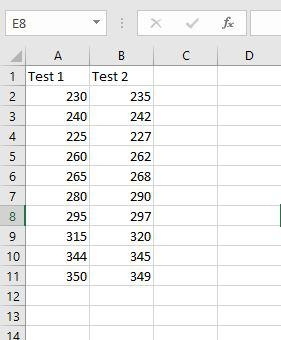Step 2: To begin, highlight all of the information, including the column headers: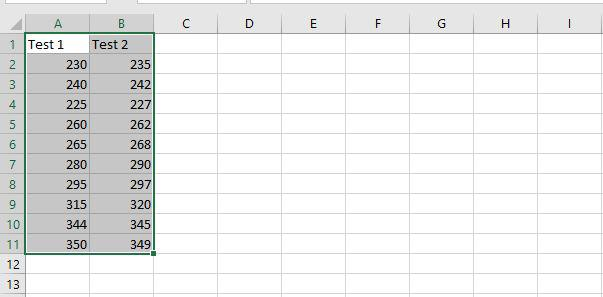Step 3: Then, select the Data tab from the top ribbon, followed by Data Analysis: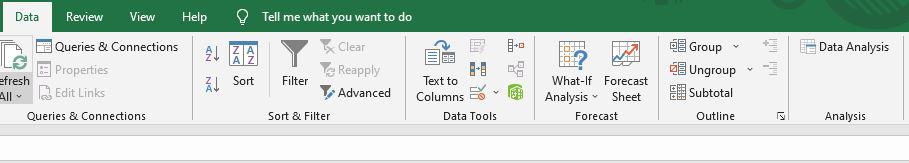Step 4: Click t-Test: paired two Samples for means and then OK in the window that displays.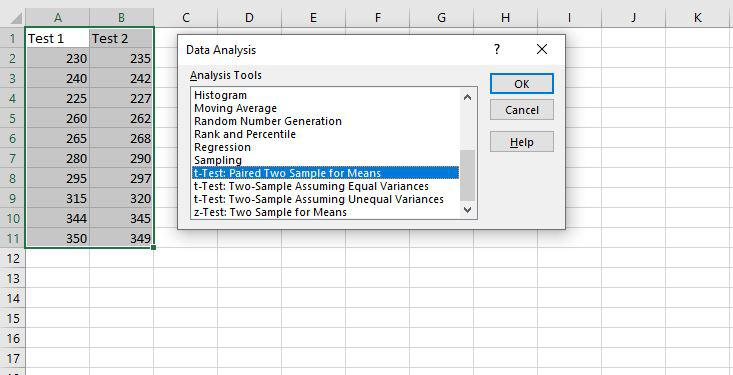Step 5: Fill in the following fields, then click OK: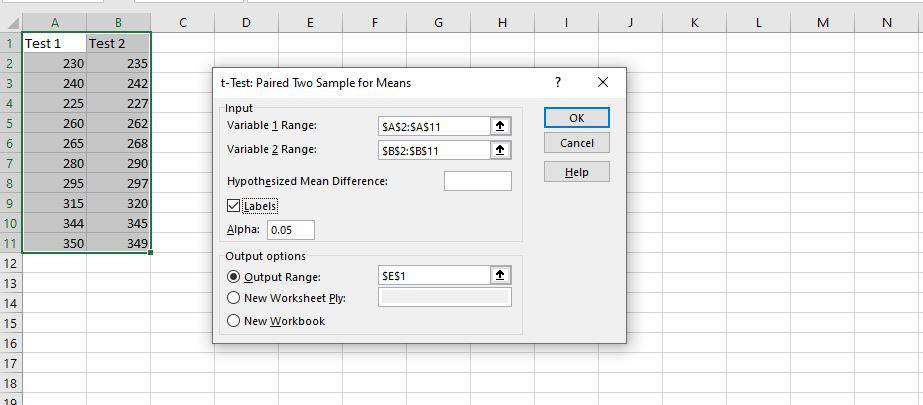Step 6: It will display a comprehensive report.This will provide the mean of each data set, its variance, the number of observations included, correlation, and P-value.

We need to look at the P-value, which is 0.02335799, which is much lower than the predicted P-value of 0.05.

Our data is significant as long as the P-value is less than 0.05.

My Personal Notes arrow_drop_up